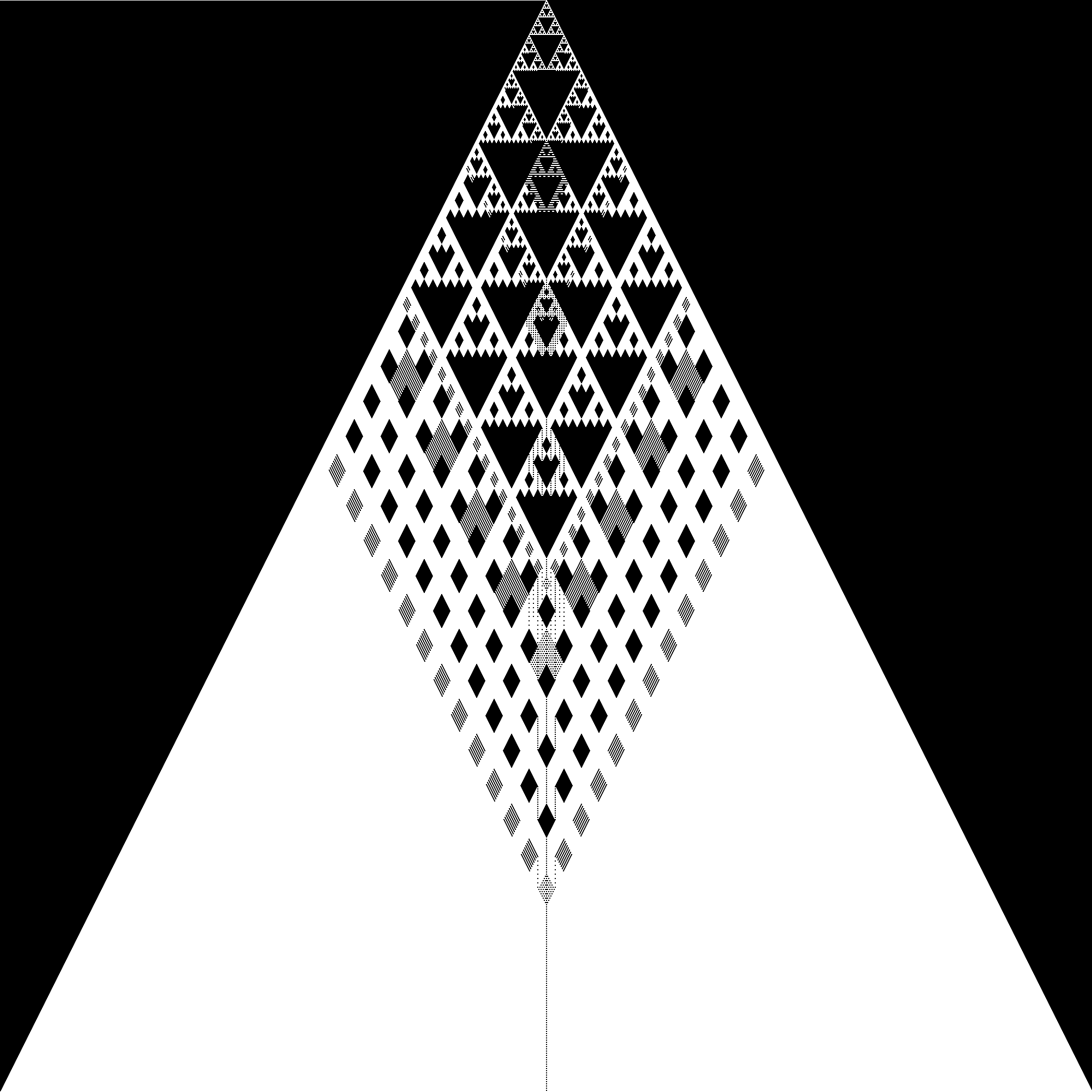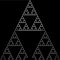# Special Divisibility

Divisibility is a ubiquitous concept. Everyone who’s attended elementary school knows what it means for one number to be divisible by another. This relatively simple concept forms the basis of many fascinating theorems, proofs, and objects in mathematics. From the prime numbers to identifying every finite abelian group up to isomorphism. However, the definition of divisibility you learned in school can be extended to create weirder and less well-understood ideas of what it means for a number to be divisible by another.

The generalization of divisibility we’ll be discussing in this article is called k-ary divisibility. k-ary divisibility extends the traditional rules of divisibility into an infinite chain of divisibility rules, each defined in terms of the previous ones. The definitions of k-ary divisibility is as follows. An integer, d, is a k-ary divisor of another integer, n, if and only if d divides n (in the traditional sense) and d and n/d share no (k-1)-ary divisors. Now, this definition may be a little confusing at first, so it may help to go through a few simple examples before moving on to more complex concepts to do with k-ary divisibility.

To start, let’s compare the traditional, unitary, and biunitary divisors of 540. Finding the traditional divisors is a simple matter of finding the prime factorization of 540, which is 2²⋅3³⋅5 (there are more factors than I want to type). Now, the prime powers in the factorization of a unitary divisor must be either 0 or equal to the corresponding power in the factorization of 540. To demonstrate why this is necessary, take d=2²⋅3². n/d=3⋅5, which shares a common traditional factor of 3 with d, therefore d cannot be a unitary divisor. Therefore, the unitary divisors of 540 are 4, 5, 27, 108, 135, and 540. To find the biunitary divisors, we follow a similar process, but instead of using the traditional divisors as a base, we use the unitary divisors. Omitting the calculations for the sake of brevity, we end up with 3, 4, 5, 9, 27, and all of their products.

We could continue on this way, naively checking every divisor of n to see if it’s a k-ary divisor of n at every step, but that’s boring. What’s more fun and useful is to find and exploit some properties of k-ary divisibility to reduce the work we need to do.

The first and most important property is that if p and q are prime powers and k-ary divisors of n, then p⋅q is also a k-ary divisor of n. If p and q are k-ary divisors of n, p and n/p share no common (k-1)-ary divisors and q and n/q also have no (k-1)-ary divisors in common. Therefore, n/(p⋅q) and p⋅q have no common (k-1)-ary divisors. This already eliminates a lot of work; we only have to check the prime power divisors of a number instead of every divisor. However, we can still do better.

It turns out that the exponents on the k-ary divisors of prime powers are the same regardless of what the prime is. To prove this, let p and q be two distinct primes and let a be the power we raise both of them to. The traditional divisors of p to the a and q to the a are p and q raised to every integral power from 0 to a, so their unitary divisors have the same powers. Now, assuming that p and q raised to the a each have the same powers on their (k-1)-ary divisors, the powers must also be the same on the k-ary divisors. Therefore, by induction the powers on the k-ary divisors of a prime power are the same regardless of the prime.

This makes finding the k-ary divisors of any integer even easier. All we need to know is the prime factorization and the powers on the k-ary factors for each prime power in that factorization. We’ve gone from needing to check every factor to only needing to check the powers on each prime power factor. We can actually make some diagrams to help out in finding k-ary divisors. We’ll make a matrix where each row and column represents a power and we’ll put a one in a given position if the power represented by that column is a k-ary divisor of the power represented by that row, otherwise we’ll put a zero. We can even turn these matrices into pictures, coloring pixels white for 1 and black for 0, and shifting the rows to make a triangle.The triangle for the 40-ary divisors

The image above is what this matrix looks like for k=40 and every power from 0 to 1,000. There seems to be an interesting pattern in the upper part of the triangle. It turns out that this pattern expands as we increase k. For example, looking at the triangle for k=447, the pattern seems to have taken up the entire triangle. It isn’t complete, as there are still some areas being filled in (the lighter areas and the missing bit at the bottom) but the framework is there.The triangle for the 447-ary divisors

You may be wondering if this pattern, which is the Sierpinski triangle for those curious, continues. Graeme L. Cohen proved it does in 1990 in his paper, On An Integer’s Infinitary divisors. As k becomes arbitrarily large, the triangle becomes arbitrarily close to the Sierpinski triangle. Additionally, for every k, there is a row, r, where every row above r in the triangle for k or any triangle after k will be identical to the Sierpinski triangle. Fortunately, if you like solving puzzles, we don’t know exactly where this row is yet. We know some bounds for where it should be, but we don’t yet know an expression for the exact position for every k.

There’s so much more to talk about with k-ary divisors that I could double the length of this post if I wanted, but I’ll leave it here for now. k-ary divisors are a relatively obscure area of mathematics, but a quick Google search will still give you plenty of reading material if you want to learn more.

Sometimes combines words and punctuation in an interesting pattern

Sometimes combines words and punctuation in an interesting pattern